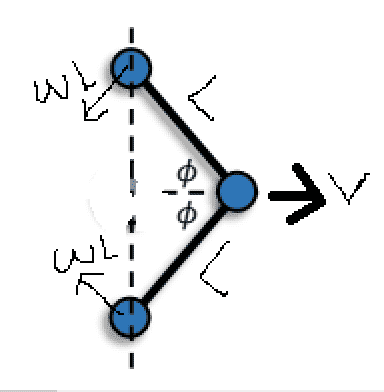# Kinetic energy of a composite body

• Rikudo
In summary, the answer to the question is that the total horizontal velocity in lab frame for the upper/lower mass is ##V_{rotation} \, sin \phi + V_{translation\,in\, x \,direction}##.f

#### Rikudo

Homework Statement
What is the total kinetic energy of this body that is made of 3 identic mass m and massless rods?
Relevant Equations
kinetic energy$$KE_{total}=KE_{centermass}+KE_{uppermass}+KE_{bottommass}$$
$$KE_{total} = \frac 1 2 (mv^2 + 2m(\vec {v} + \vec {wL})^2)$$

But, the solution manual says that the answer is this:
$$KE_{total} = \frac 1 2 (mv^2 + 2m(v^2+w^2L^2))$$

I think he regard this composite body as an ordinary rigid body (e.g a pancake),However, the angular velocity of the upper and lower mass has different direction. So, I doubt that this is correct.

What do you think?

Last edited:
•PeroK
I agree with your answer, but not your argument concerning the different directions of the upper and lower masses.
Consider ##\phi=\pi/2##. At this point, those two masses are not moving, so have no KE. ##\vec v+\vec\omega L=0##.

Btw, you forgot the power of 2 on omega in your typing of the book answer.

•Rikudo
Btw, you forgot the power of 2 on omega in your typing of the book answer.
Ah..yes. thank you for reminding me.

The exact setup is unclear to me. Is the entire composition moving at ##\vec v## and on top of that the masses to the left have an additional angular velocity ##\omega##?

The exact setup is unclear to me. Is the entire composition moving at ##\vec v## and on top of that the masses to the left have an additional angular velocity ##\omega##?
yes

Ok, so here is the thing. In general of course the total kinetic energy of a collection of ##n## masses ##m_i## with respective velocities ##\vec v_i## is given by
$$K = \sum_{i = 1}^N \frac{m_i}{2} \vec v_i^2.$$
It can be shown that this is equal to
$$K = \sum_{i = 1}^N \frac{m_i}{2} (v^2 + u_i^2)$$
where ##\vec u_i## is the velocity of mass ##i## relative to the center of mass and ##v## is the speed of the center of mass. However, this split is only possible relative to the center of mass, not generally relative to any other point. The given solution seems to misapply this formula as ##\vec v## is not the center of mass velocity, but the velocity of a particular mass.

Last edited:
•topsquark, PeroK and malawi_glenn
Just to solidify the above, the definition of the center of mass velocity is
$$\vec v = \frac{1}{M} \sum_{i=1}^N m_i \vec v_i$$
where ##M## is the sum over the masses. By definition ##\vec v_i = \vec v + \vec u_i## and so
$$K = \sum_{i=1}^N \frac{m_i}{2} (\vec v + \vec u_i)^2 = \sum_{i=1}^N \frac{m_i}{2} (v^2 + 2\vec v\cdot \vec u_i + u_i^2) = \frac{M}{2} v^2 + \vec v\cdot \sum_{i=1}^N m_i \vec u_i + \sum_{i=1}^N \frac{m_i}{2} u_i^2.$$
However,
$$\sum_{i=1}^N m_i \vec u_i = \sum_{i = 1}^N m_i(\vec v_i - \vec v) = \sum_{i=1}^N m_i \vec v_i - M\vec v = 0$$
and so
$$K = \frac{M}{2} v^2 + \sum_{i=1}^N \frac{m_i}{2} u_i^2 = \sum_{i=1}^N \frac{m_i}{2}(v^2 + u_i^2).$$
The step that fails if we replace the center of mass velocity ##\vec v## by any other velocity ##\vec w## is that the sum
$$\sum_{i=1}^N m_i \vec v_i \neq M\vec w$$
and therefore the cross term does not disappear.

Last edited:
•topsquark, PeroK and malawi_glenn
the entire composition moving at ##\vec v## and on top of that the masses to the left have an additional angular velocity ##\omega##?

If initially the system is in a straight line (##\phi = 90 ^\circ##) and at rest, then we struck the mass at the center so that it has velocity ##v_o## to the right, after some time (when the velocity of the mass at the center is ##v##), will the upper and lower masses' translation velocities in horizontal direction also be ##v##?

If initially the system is in a straight line (##\phi = 90 ^\circ##) and at rest, then we struck the mass at the center so that it has velocity ##v_o## to the right, after some time (when the velocity of the mass at the center is ##v##), will the upper and lower masses' translation velocities in horizontal direction also be ##v##?
That depends on the properties of the body and the constraints that are put on the angle ##\phi##. If the system is fully rigid, then all three masses will get the same velocity immediately. If the side masses are free to rotate (allowing any ##\phi##) then they will keep rotating around the middle mass.

They are free to rotate.

The total horizontal velocity in lab frame for the upper/lower mass is ##V_{rotation} \, sin \phi + V_{translation\,in\, x \,direction}##.

But, is the translation velocity always the same with the middle mass's?

They are free to rotate.

The total horizontal velocity in lab frame for the upper/lower mass is ##V_{rotation} \, sin \phi + V_{translation\,in\, x \,direction}##.

But, is the translation velocity always the same with the middle mass's?
No.

•Rikudo
No.
There may be a misunderstanding here. @Rikudo wrote
The total horizontal velocity in lab frame for the upper/lower mass is ##V_{rotation} \, sin \phi + V_{translation\,in\, x \,direction}##.
but did not define ##V_{translation\,in\, x \,direction}. Based on that equation, he is taking it as the velocity of the middle mass.